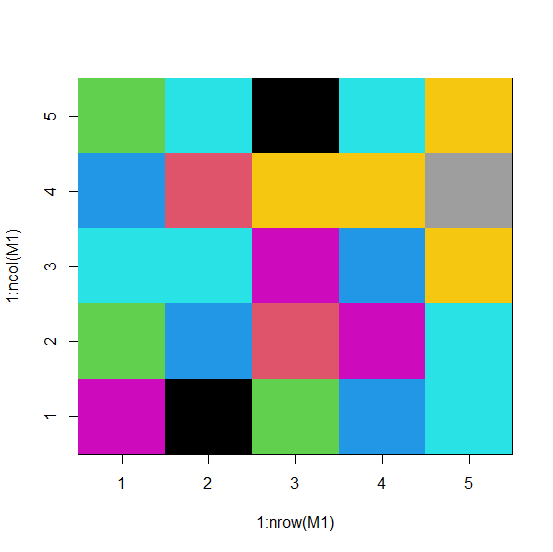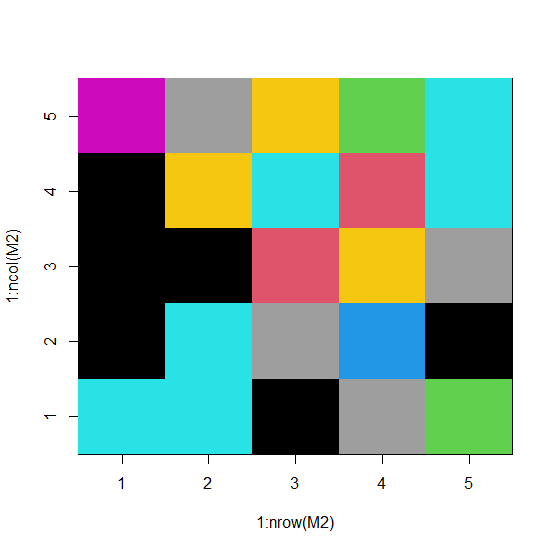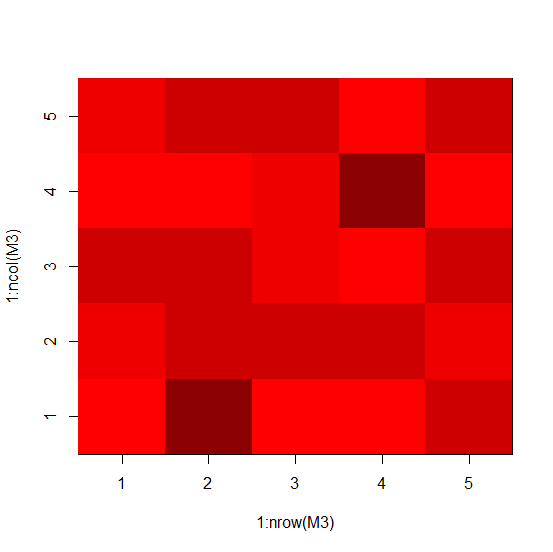# How to convert a matrix into a color matrix in R?

To convert a matrix into a color matrix, we can make use of image function. There are multiple ways for assigning the colors but the easiest one might be by defining the minimum and maximum value in the matrix. Also, we can do this by using the shades of a single color as shown in the example 3.

## Example1

Live Demo

> M1<-matrix(rpois(25,5),ncol=5)
> M1

## Output

   [,1] [,2] [,3] [,4] [,5]
[1,] 6    3    5    4    3
[2,] 9    4    5    2    5
[3,] 3    2    6    7    9
[4,] 4    6    4    7    5
[5,] 5    5    7    8    7

## Example

> image(1:nrow(M1),1:ncol(M1),M1,col=min(M1):max(M1))

## Output## Example2

Live Demo

> M2<-matrix(sample(0:9,25,replace=TRUE),nrow=5)
> M2

## Output

   [,1] [,2] [,3] [,4] [,5]
[1,] 5    1    1    9    6
[2,] 5    5    1    7    8
[3,] 1    8    2    5    7
[4,] 8    4    7    2    3
[5,] 3    1    8    5    5

## Example

> image(1:nrow(M2),1:ncol(M2),M2,col=min(M2):max(M2))

## OutputExample3

We can also do it by defining the colors −

Live Demo

> M3<-matrix(sample(0:4,25,replace=TRUE),nrow=5)
> M3

## Output

   [,1] [,2] [,3] [,4] [,5]
[1,] 0    2    3    0    2
[2,] 4    3    3    1    3
[3,] 1    3    2    2    3
[4,] 1    3    0    4    0
[5,] 3    2    3    0    3

## Example

> colors<-c("0"="red","1"="red1","2"="red2","3"="red3","4"="red4")
> image(1:nrow(M3),1:ncol(M3),M3,col=colors)

## Output